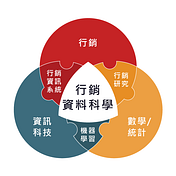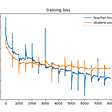# 一、如果要深入資料科學，可以透過什麼資源來了解呢?

Q12. Who/what are your favorite media sources that report on data science topics? (Select all that apply) — Selected Choice

Q13. On which platforms have you begun or completed data science courses? (Select all that apply) — Selected Choice

## 1. 喜愛資源資料預處理

`# 取出Q12的資料lst = []for i in multiple_choice.columns:    if i[:3] == 'Q12':        lst.append(i)source = multiple_choice[lst].iloc[1:,]# 取出Q12多選題的選項choose =  multiple_choice[lst].iloc.apply(lambda x: ''.join(x.split('-')[2:]).replace(' ',''))# 統計各選項選擇數量source_df = pd.DataFrame()source_df['choice'] = list(choose.values)source_df['value'] = list(map(lambda i: len(source.iloc[:,i].dropna()), list(range(source.shape))))source_df`

## 2. 喜愛課程資料預處理

`# 取出Q13的資料lst = []for i in multiple_choice.columns:    if i[:3] == 'Q13':        lst.append(i)course = multiple_choice[lst].iloc[1:,]# 取出Q13多選題的選項choose =  multiple_choice[lst].iloc.apply(lambda x: ''.join(x.split('-')[2:]).replace(' ',''))# 統計各選項選擇數量course_df = pd.DataFrame()course_df['choice'] = list(choose.values)course_df['value'] = list(map(lambda i: len(course.iloc[:,i].dropna()), list(range(course.shape))))course_df['choice'] = course_df['choice'].replace('KaggleCourses(i.e.KaggleLearn)','KaggleCourses')course_df['choice'] = course_df['choice'].replace('UniversityCourses(resultinginauniversitydegree)','UniversityCourses')course_df`

## 3. 喜愛資源資料視覺化

`# 圖表繪製import plotly.express as pxfig = px.pie(source_df.iloc[:-3,], values='value', names='choice',   　　　　　title='Media Sources Selected',　　　　　color_discrete_sequence=px.colors.sequential.Sunsetdark)fig.update_traces(textposition='inside')fig.show()# 自動儲存pyplt(fig, filename ='Media Sources Selected.html')`

## 4. 喜愛課程資料視覺化

`# 圖表繪製import plotly.express as pxfig = px.pie(course_df.iloc[:-3,], values='value', names='choice',              title='Data Science Courses Selected',             color_discrete_sequence=px.colors.sequential.Mint)fig.update_traces(textposition='inside', textinfo='percent+label')fig.show()# 自動儲存pyplt(fig, filename ='Data Science Courses Selected.html')`

## 5. 分析

• Kaggle (forums, blog, social media, etc.)
• Blogs (TowardsDataScience, Medium, AnalyticsVidhya, KDnuggets, etc.)

# 來幫大家結論一下~

• 資料科學家主要工作內容有哪些?
分別為「分析並了解資料對產品或企業決策的影響、探索機器學習應用領域、改善現有的機器學習模型」簡單地來說，只要掌握好數理、資訊科學、應用領域三方面的技能，資料科學家的工作絕對難不倒你!
• 資料科學家會使用哪種程式語言?
Python近乎完勝所有工作領域，因此會建議新手從Python開始學起。而各個程式語言的功能也有所不同，可以根據資料處理的需求來選擇最適合的工具。此外，也非常推薦學習R語言，在資料分析中的統計分析及視覺化上可以大大助您一臂之力!
• 如果要深入資料科學，可以透過什麼資源來了解呢?
首先，我建議可以從Udemy的Machine learning A-Z課程來入門。在了解Python的基礎後，推薦觀看台大李宏毅教授的機器學習課程也可以根據自己的需求或有興趣的領域，在CourseraKaggleCoursesUdemy平台上選擇最適合的課程!

# Plotly套件補充資訊!

1. Dynamic & Interactive
2. Open-source plotting library
3. Supports over 40 unique chart types
4. Covering a wide range of statistical, financial, geographic, scientific, and 3-dimensional use-cases
5. Support for Python and R

## 1. 資料預處理

`# 抓取Q3、Q5、Q5，並去除空值及符號world_salary = multiple_choice[['Q3','Q5','Q10']].iloc[1:,:]world_salary = world_salary.dropna()world_salary['Q10'] = world_salary['Q10'].str.replace('\$','', regex=False)world_salary['Q10'] = world_salary['Q10'].str.replace(',','', regex=False)world_salary`
`# 薪水的上、下區間world_salary['salary_lower_bound'] = pd.to_numeric(world_salary['Q10'].str.split('-', expand=True), errors='coerce')world_salary['salary_upper_bound'] = pd.to_numeric(world_salary['Q10'].str.split('-', expand=True), errors='coerce') + 1# 取上、下區間中間值world_salary['salary'] = (world_salary['salary_upper_bound'] + world_salary['salary_lower_bound'])/2# 更改column namesworld_salary.columns = ['country', 'job', 'salary_range', 'salary_lower_bound', 'salary_upper_bound', 'salary']world_salary`
`# 取出資料科學家的資料world_salary_ds = world_salary[world_salary['job'] == 'Data Scientist']# 計算國家的平均薪水country_salary_ds = pd.DataFrame(world_salary_ds.groupby('country')['salary'].mean().astype('int'))country_salary_ds = country_salary_ds.reset_index()country_salary_ds = country_salary_ds.sort_values(by = 'salary', ascending = False).reset_index(drop = True)# 更改國家名稱country_salary_ds['country'] = country_salary_ds['country'].str.replace('United States of America','United States')country_salary_ds['country'] = country_salary_ds['country'].str.replace('United Kingdom of Great Britain.*','United Kingdom')country_salary_ds['country'] = country_salary_ds['country'].str.replace('Iran, Islamic Republic.*','Iran')country_salary_ds['country'] = country_salary_ds['country'].str.replace('South Korea','Korea, Rep.')country_salary_ds['country'] = country_salary_ds['country'].str.replace('Republic of Korea','Korea, Dem. Rep.')country_salary_ds['country'] = country_salary_ds['country'].str.replace('Hong Kong (S.A.R.)','Hong Kong, China')country_salary_ds['country'] = country_salary_ds['country'].str.replace('Viet Nam','Vietnam')country_salary_ds.head(10)`
`#加入country codeimport plotly.express as pxdf = px.data.gapminder().query("year == 2007")[['country','continent','iso_alpha']]country_salary_merge_ds = pd.merge(country_salary_ds, df, on = 'country')# 將缺少的國家加入country_salary_merge_ds = country_salary_merge_ds.append({'country':'Russia','salary':28000,'continent':'Europe','iso_alpha':'RUS'},ignore_index=True)country_salary_merge_ds = country_salary_merge_ds.append({'country':'Ukraine','salary':29102,'continent':'Europe','iso_alpha':'UKR'},ignore_index=True)country_salary_merge_ds = country_salary_merge_ds.append({'country':'Belarus','salary':27568,'continent':'Europe','iso_alpha':'BLR'},ignore_index=True)country_salary_merge_ds.head(10)`

## 2. 資料視覺化

`# 圖表繪製import plotly.express as pxfig = px.scatter_geo(country_salary_merge_ds, locations="iso_alpha",size="salary", color="salary", hover_name="country",projection="natural earth",title = 'Average Data Scientists Salary For Every Countries')fig.show()# 自動儲存pyplt(fig, filename ='Average Data Scientists Salary For Every Countries.html')`

# 2020課程地圖

--

--

--

## More from 行銷資料科學

Love podcasts or audiobooks? Learn on the go with our new app.## Implementing Meta Pseudo Labels — Part 3 — Losses, Training and Evaluating## Has WWE Finally Moved On From The Nostalgia Era? (2/2)## Bigcommerce Dynamics AX Integration Connector## Data Cleansing made Simple using SimpleData Management